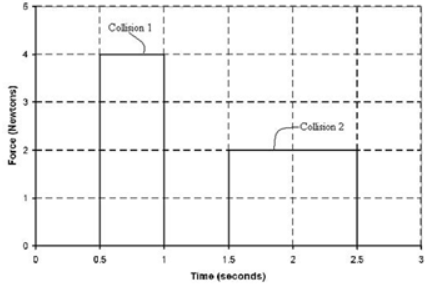# Problem: The force vs. time graphs for two collisions are shown. Which collision has the largest impulse? A. The impulses are the same. B. The impulse for collision 2 since it lasts longer. C. The impulse for collision 1 since it has the larger maximum force. D. Cannot be determined since the initial an final speeds are unknown. E. Cannot be determined since the masses are unknown.

###### FREE Expert Solution

For varying forces, the total impulse is the area under the Force-Time graph.

$\overline{){\mathbf{J}}{\mathbf{=}}{\mathbf{Area}}{\mathbf{\left[}}{\mathbf{F}}{\mathbf{\left(}}{\mathbf{t}}{\mathbf{\right)}}{\mathbf{\right]}}}$

81% (206 ratings)###### Problem Details

The force vs. time graphs for two collisions are shown. Which collision has the largest impulse?A. The impulses are the same.

B. The impulse for collision 2 since it lasts longer.

C. The impulse for collision 1 since it has the larger maximum force.

D. Cannot be determined since the initial an final speeds are unknown.

E. Cannot be determined since the masses are unknown.